21 is a popular number in this quiz!

# Fractions (Dividing)

This Math quiz is called 'Fractions (Dividing)' and it has been written by teachers to help you if you are studying the subject at middle school. Playing educational quizzes is a fabulous way to learn if you are in the 6th, 7th or 8th grade - aged 11 to 14.

It costs only \$12.50 per month to play this quiz and over 3,500 others that help you with your school work. You can subscribe on the page at Join Us

If you’ve tackled addition and subtraction and then multiplication of fractions, it’s now time to start dividing. Like multiplication, dividing fractions is rather easy once you understand the simple placement of your numbers.

When you tried to simplify your fractions in multiplication, you learned that you had to use division. For example, let’s look at one of the multiplication problems given in an earlier quiz. See below.

For each division problem below, find the correct solution/answer.

Go straight to Quiz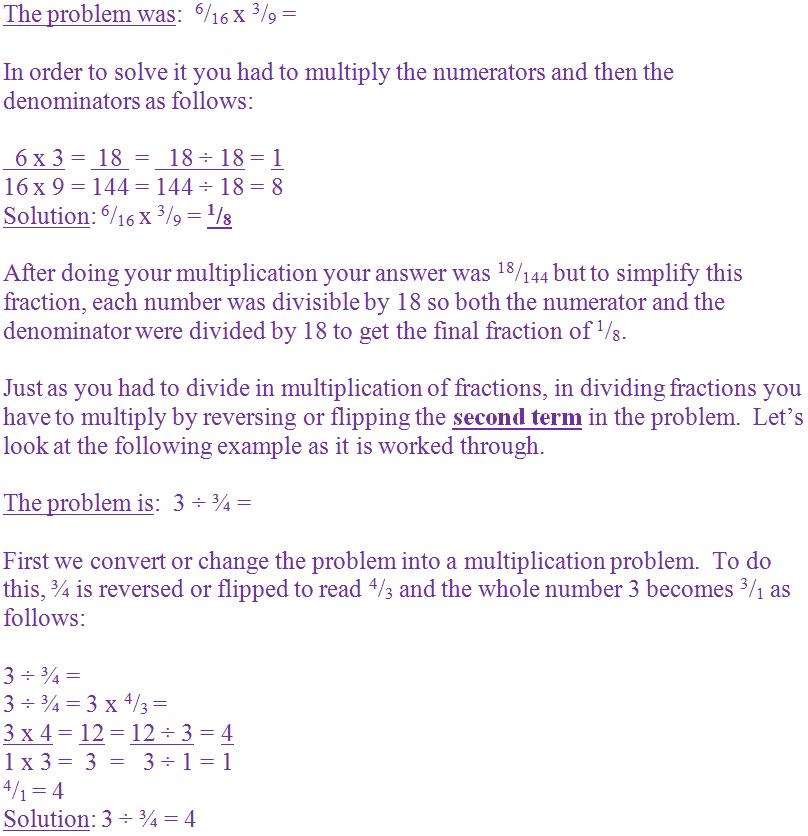1.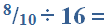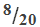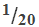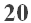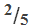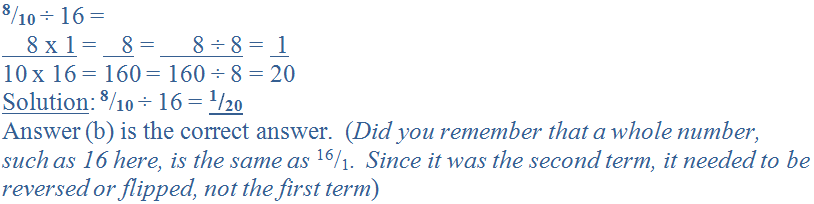2.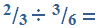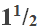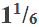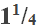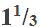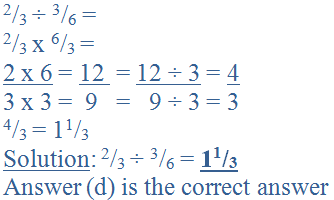3.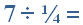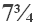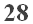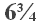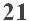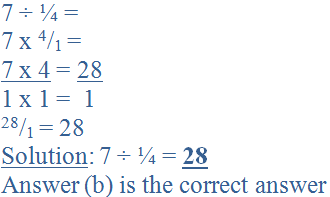4.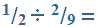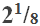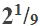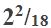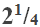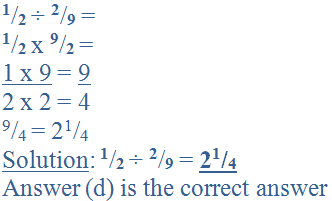5.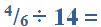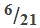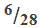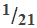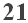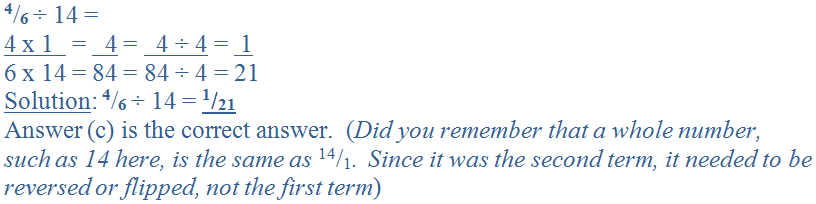6.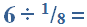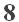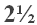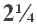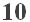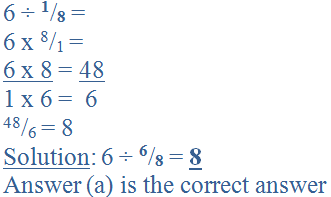7.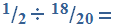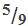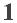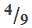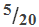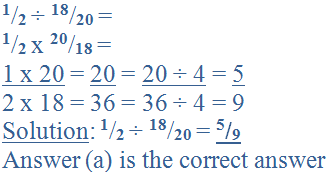8.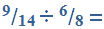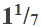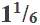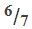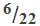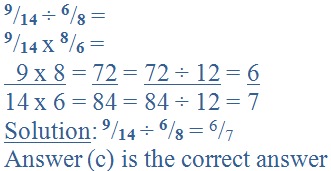9.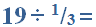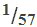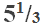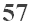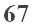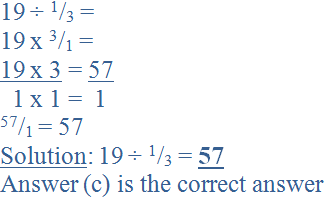10.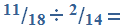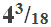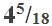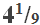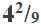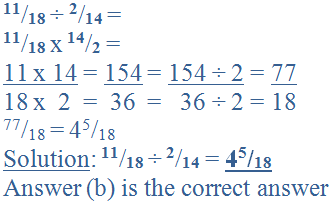Author:  Christine G. Broome